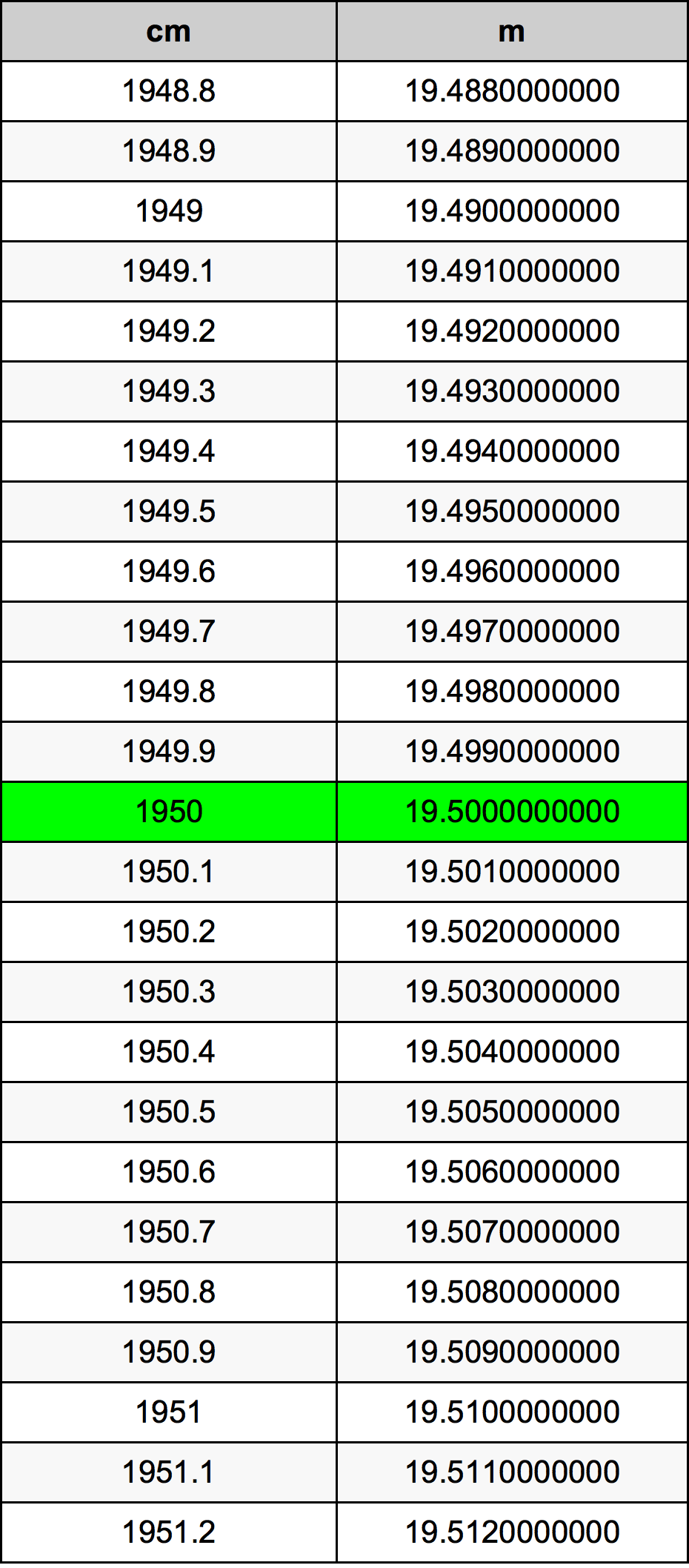Cm To M

# 1950 cm to m1950 Centimeters to Meters

cm
=
m

## How to convert 1950 centimeters to meters?

 1950 cm * 0.01 m = 19.5 m 1 cm
A common question is How many centimeter in 1950 meter? And the answer is 195000.0 cm in 1950 m. Likewise the question how many meter in 1950 centimeter has the answer of 19.5 m in 1950 cm.

## How much are 1950 centimeters in meters?

1950 centimeters equal 19.5 meters (1950cm = 19.5m). Converting 1950 cm to m is easy. Simply use our calculator above, or apply the formula to change the length 1950 cm to m.

## Convert 1950 cm to common lengths

UnitLengths
Nanometer19500000000.0 nm
Micrometer19500000.0 µm
Millimeter19500.0 mm
Centimeter1950.0 cm
Inch767.716535433 in
Foot63.9763779528 ft
Yard21.3254593176 yd
Meter19.5 m
Kilometer0.0195 km
Mile0.0121167382 mi
Nautical mile0.0105291577 nmi

## What is 1950 centimeters in m?

To convert 1950 cm to m multiply the length in centimeters by 0.01. The 1950 cm in m formula is [m] = 1950 * 0.01. Thus, for 1950 centimeters in meter we get 19.5 m.

## 1950 Centimeter Conversion Table## Alternative spelling

1950 Centimeter to Meter, 1950 Centimeter in Meter, 1950 Centimeters to Meters, 1950 Centimeters in Meters, 1950 Centimeters to m, 1950 Centimeters in m, 1950 cm to m, 1950 cm in m, 1950 Centimeter to Meters, 1950 Centimeter in Meters, 1950 Centimeter to m, 1950 Centimeter in m, 1950 Centimeters to Meter, 1950 Centimeters in Meter